# Limits of functions

Limit of a function. Some remarkable limits.
Infinitesimal and infinite values. Finite limit.
Infinite limit. Notion of an infinity.

Limit of a function. A number L is called a limit of a function  y = f ( x ) as  x  tends  a :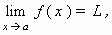if and only if for any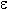> 0 one can find such a positive number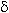=(), depending on, that | x a | <implies that | f ( x ) – L | <.

This definition means, that L is a limit of a function y = f ( x ) , if the function value approaches unrestrictedly to L , when the argument value x approaches a . Geometrically it means, that for any> 0 it is possible to find such a number, that if x is within an interval ( a, a +), then a function value is within an interval ( L, L +). Note, that according to this definition, a function argument x only approaches a , not adopting this value! It must be considered at calculating limit of any function at a point of its discontinuity ( i.e., where this function doesn’t exist ).

E x a m p l e .  Find :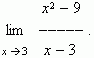S o l u t i o n .  Substituting x = 3  into the expression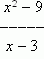we’ll receive a meaningless
expression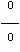( see "About meaningless expressions" in the section "Powers and roots"
of the part "Algebra"). Therefore we’ll solve in a different way: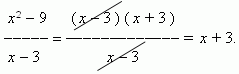Here the fraction canceling is valid, because x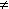3 , it only approaches 3.
Now we have: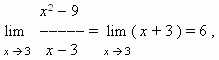because, if x approaches  3 ,  then x + 3  approaches  6 .

Some remarkable limits.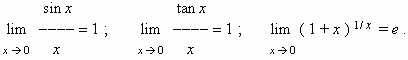Infinitesimal and infinite value. If limit of some variable is equal to 0, this variable is called an infinitesimal .

E x a m p l e .  The function y =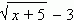is an infinitesimal, if x approaches  4, because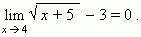If an absolute value of some variable increases unboundedly, then this variable is called an infinite value .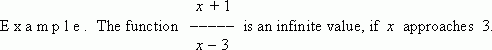An infinite value has no a finite limit, but it has so called an infinite limit; this fact is written as: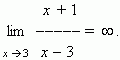The symbol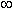("infinity") doesn’t mean some number, it means only that the fraction increases unboundedly if x approaches 3. It should be noted, that the fraction can be both positive (at x > 3) and negative (at x < 3). If an infinite value can be only positive at any values of x ,  it is marked in a record. For example, at x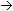0  the function y = x –2 is an infinite value, but it is positive both at x > 0 and at x < 0 ; this is expressed as: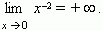On the contrary, the function y = – x –2 is always negative, therefore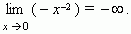According to this, a result of our example can be written as: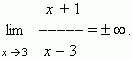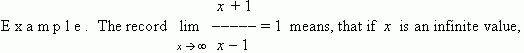then this fraction approaches 1 , i.e. its limit is 1.